# Light, Phase and Group Velocities

If any of you experts see any problems with the math, please get in touch with us, we want this to be 100% correct.

## Velocity of light

By now you should have memorized the speed of light in a free space ("c"), it's 2.9979E8 meters second, or about 186,000 miles per second, or as a rule of thumb, about one foot per nanosecond. This is the speed limit of the universe; no matter or information is allowed to travel faster.

Speaking of "c", we recommend NOT to hire a microwave engineer candidate who doesn't know the velocity of light, permeability and permittivity of free space. Go to our table of physical constants and commit them to memory. And don't fall for this trick question on a quiz:

Question: what's the speed of light

Correct answer: it depends on the medium!

Note to Microwaves101 readers: most textbooks use the term "phase velocity" denoted by vp interchangeably to also mean "velocity of light in a medium". This gets confusing, so we will avoid doing it and denote "velocity of light in a medium" by vlight.

The velocity of light is only a function of the permeability and permittivity of the medium: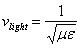In free space, the values for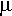0 and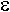0 are used, and the speed is denoted by the constant "c":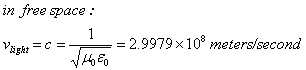### Velocity of light from lumped transmission line model

Before we reveal this knowledge, let us point out that the lumped transmission line model now has its own page!

Velocity of light in a transmission line can be derived from the inductance and capacitance per unit length. Going back to our lumped element transmission line model...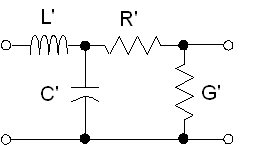Under the normal conditions of: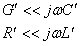The lumped model reduces to: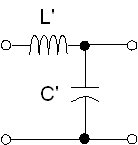The velocity of light in the transmission line is simply: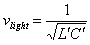Note the similarity to this equation:### Velocity factor

In a media other than free space, light is slowed down, never sped up. We can define a velocity factor "VF" to account for this. The velocity factor will always be less than unity, remember that the speed of light in vacuum is the speed limit of the universe.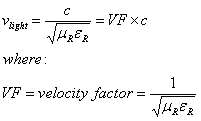Most media that we are interested in have relative permeability=1, therefore the velocity factor is simply: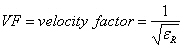Typically, coax vendors will quote a velocity factor for their wares. If the "stuffing" in a coax cable is PTFE, with a dielectric constant (relative permittivity) of 2.07, the velocity factor is 69.5%.

Velocity of light for quasi-TEM transmission lines such as microstrip and coplanar waveguide is equal to the speed of light divided by the square root of the effective relative permittivity.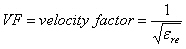For a description of what is meant by effective relative permittivity, go here. Sometimes it is denoted as keff, sometimesre.

Frequency doesn't change when a signal encounters material and transmission line effects, but wavelength does. The change in wavelength compared to free space is inversely proportional to velocity factor.

## Phase velocity

The phase velocity is the speed at which a point of fixed phase propagates, which is not always the speed that electromagnetic information travels. In TEM waves, the phase velocity is the same as the velocity of light in the chosen medium.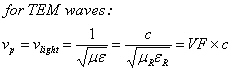For waveguide, the phase velocity is more complicated: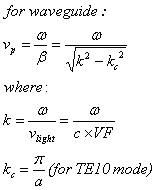After some rearranging we can arrive at: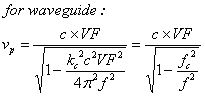We like the more complicated form of the equation on the left, because it has been reduced to a function of velocity factor VF and cutoff wavenumber which relate to dimensions and material properties. Here you can see that the velocity factor has two effects: it directly reduces the phase velocity in the numerator, while its placement in the denominator acts to increase phase velocity. The form of the equation on the right, the velocity factor term in the denominator is built in to the cutoff frequency term fc. From this you can see that when the cutoff frequency is reached, the phase velocity goes to infinity.

For air-filled waveguide, the phase velocity reduces further to: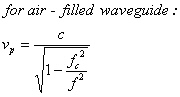## Group velocity

Group velocity is the speed at which electromagnetic information travels. Group delay is the length of a circuit or transmission line, divided by its group velocity. Although we aren't smart enough to bore you with the details of how this is derived (order a copy of Pozar from our book page), group velocity is slowed by the inverse of the factor that phase velocity is increased. In the general case,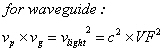Another way to express this is: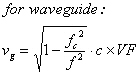It can be seen that this implies that group velocity is less than the speed of light, while phase velocity is greater than the speed of light.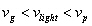Going back to the case of air-filled waveguide, the group velocity is: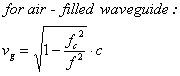The inequality of group velocity, speed of light in vacuum, and phase velocity is then: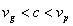Author : Unknown Editor